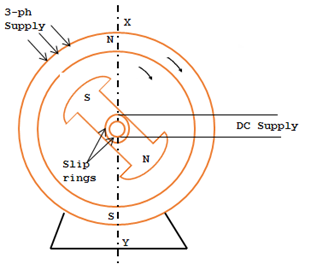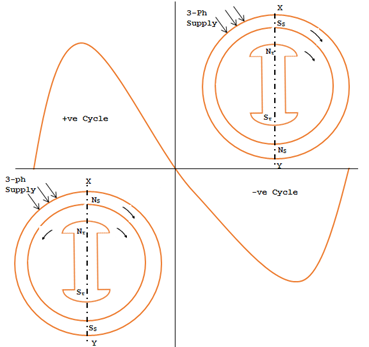# Principle of Operation of Synchronous Motor

### Introduction:

A Synchronous Motor is a constant speed AC motor. It takes AC power by the stator from mains supply for its working in addition it utilizes a small amount of DC power which is fed to its Rotor, we need two kinds of power sources that is one is AC and another is DC as shown in the fig below.Some important characteristic of Synchronous motor:
1. It runs at a constant speed (i.e) at synchronous speed determined by the number poles and the frequency or not at all. The only way to change its speed is to vary the supply frequency.
2. It is capable of being operating under a wide range of power factors such in lagging, leading and unity. Hence it can be used for power factor correction purposes in addition to supplying torque to drive loads.
3. The main drawback of synchronous motor is that it is not self-starting. Hence it has to be run up to synchronous speed by some external means before it can be connected to the supply. This drawback is ignored, considering its constant and variable power factor working condition.

### Construction:

A synchronous motor is identical in construction with an AC generator or alternator. The synchronous motor has a stator and rotor. A three phase winding is placed in the stator which draws current from an AC source and produces a Rotating Magnetic Field.
Rotor consists of electromagnetic Poles. The construction of the rotor can be salient pole type or non-salient pole type. The rotor pole draws the current from a DC source and gives a DC electromagnetic field, for locking with the rotating magnetic field.

### Principle of Operation:

If a three phase AC supply is given to a three phase stator winding of the motor, a rotating magnetic field is produced. This can be considered as a north and South Pole rotating in space at Synchronous Speed as shown in the fig. The two stator poles are assumed and marked as Ns and SS. They are assumed to rotate in clockwise direction at synchronous speed.

Synchronous Speed Ns = 120f/P
Where f = frequency of the supply
P = No of poles in the stator.

DC excitation to the rotor which forms the rotor poles Nr and Sr. At an instant for the rotor position indicated in figure the stator poles are at points X is Ns and Y is SS.Now there is a reaction between stator and rotor poles. Like poles NS and Nr of stator and rotor repel each other. Because the stator field moves in clockwise direction, Nr and Sr of the rotor tends to rotate in the anticlockwise direction.

Half a cycle later the position of the stator poles are interchanged due to RF. This reverse of stator polarity is very rapid. Ns is at point Y and SS is at point X. Now Ns attracts Sr and Ss attracts Nr. The rotor now tends to rotate in clockwise thus the rotor is faced with a torque which is changing in quick succession in every half cycle. First the rotor begin to move in anti-clockwise direction and then in clockwise direction, at every half a cycle. Due to inertia, the rotor cannot move in any direction and becomes stationary. Therefore the synchronous motor has no starting torque and cannot start its own.This post first appeared on My Tech Info, please read the originial post: here

# Share the post

Principle of Operation of Synchronous Motor

×